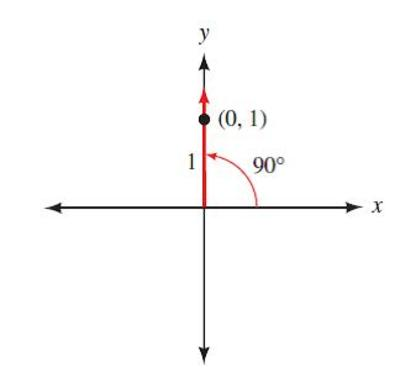## Trigonometry 7th Edition

To find out the value of $\csc 90^{\circ}$- We will draw $90^{\circ}$ in standard position, locate point (0.1) on the terminal side and use Definition I- Using the point (0,1) x = 0, y = 1 and r = 1 $\csc 90^{\circ} = \frac{r}{y}$ $\csc 90^{\circ} = \frac{1}{1}$ $\csc 90^{\circ}$ = 1